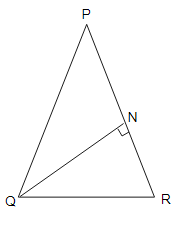# In a triangle $P Q R, N$ is a point on $P R$ such that $Q N \perp P R$. If $P N$. $N R=Q^{2}$, prove that $\angle \mathrm{PQR}=90^{\circ}$.

Given:

In a triangle $P Q R, N$ is a point on $P R$ such that $Q N \perp P R$.

$P N$. $N R=Q^{2}$

To do:

We have to prove that $\angle \mathrm{PQR}=90^{\circ}$.

Solution:$P N . N R=Q N^{2}$

$P N . N R=Q N . Q N$

This implies,

$\frac{P N}{Q N}=\frac{Q N}{N R}$...........(i)

In $\triangle QNP$ and $\triangle RNQ$,

$\frac{P N}{Q N}=\frac{Q N}{N R}$

$\angle P N Q=\angle R N Q$

Therefore, by SAS similarity,

$\angle Q N P \sim \angle R N Q$

This implies,

$\angle P Q N=\angle Q R N$........(ii)

$\angle R Q N=\angle Q P N$........(iii)

Adding (ii) and (iii), we get,

$\angle P Q N+\angle R Q N=\angle Q R N+\angle Q P N$

$\angle P Q R=\angle Q R N+\angle Q P N$

We know that,

Sum of the angles of a triangle is $180^{\circ}$.

In $\triangle P Q R$,

$\angle P Q R+\angle Q P R+\angle Q R P=180^{\circ}$

$\angle P Q R+\angle Q P N+\angle Q R N=180^{\circ}$

$\angle P Q R+\angle P Q R=180^{\circ}$

$2 \angle P Q R=180^{\circ}$

$\angle P Q R=90^{\circ}$

Hence proved.

Updated on: 10-Oct-2022

60 Views